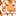# QlikView App Dev

Discussion Board for collaboration related to QlikView App Development.

cancel
Showing results for
Did you mean:Creator II

## SET ANALYSIS

Dear Community ,

i want  two expression with set analysis -

1-  sum of "amount" column  with " condition is "account_code" = 4332

2- sum of "amount" with condition is "account_code" = 3301,3302,3303,3304. plz revert  so can get the correct value

6 RepliesSpecialist

=Sum({<account_code = {"4332"}>}amount)

=Sum({<account_code = {"3301","3302","3303","3304"}>}amount)MVP

Hi,

1:

sum({<account_code={"4332"}>}amount)

2:

sum({<account_code={"3301","3302","3303","3304"}>}amount)

Regards

Great dreamer's dreams never fulfilled, they are always transcended.Master III

hi try this

1. sum({<account_code={'4332'}>}amount)

2. sum({<account_code={'3301','3302','3303','3304'}>}amount)Master III

=sum({<account_code = {4332}>}amount)

=sum({<account_code = {3301,3302,3303,3304}>}amount)Specialist III

=Sum({\$<account_code={'4332'}>}amount)

=Sum({\$<account_code={'3301','3302','3303','3304'}>}amount)MVP

Hi,

Please find detailed set analysis syntax and examples using this document you can write your own expression.

VikasCommunity Browser